## 20141128

### Astronomy current events question: CIBER observations of cosmic light

Astronomy 210L, fall semester 2014
Cuesta College, San Luis Obispo, CA

Students are assigned to read online articles on current astronomy events, and take a short current events quiz during the first 10 minutes of lab. (This motivates students to show up promptly to lab, as the time cut-off for the quiz is strictly enforced!)
Kathy Svitil, "Caltech Rocket Experiment Finds Surprising Cosmic Light," (November 6, 2014)
http://www.caltech.edu/content/caltech-rocket-experiment-finds-surprising-cosmic-light
CalTech's Cosmic Infrared Background Experiment (CIBER) mission used suborbital rockets to observe light from:
(A) matter falling into supermassive black holes.
(B) stray stars between galaxies.
(C) the aurora borealis.
(D) active solar flares.
(E) volcanic greenhouse gas emissions.

Student responses
Sections 70178, 70186
(A) : 6 students
(B) : 21 students
(C) : 4 students
(D) : 6 students
(E) : 1 student

### Astronomy current events question: Philae lander in hibernation

Astronomy 210L, fall semester 2014
Cuesta College, San Luis Obispo, CA

Students are assigned to read online articles on current astronomy events, and take a short current events quiz during the first 10 minutes of lab. (This motivates students to show up promptly to lab, as the time cut-off for the quiz is strictly enforced!)
European Space Agency press release, "Pioneering Philae Completes Main Mission before Hibernation," (November 15, 2014)
http://www.esa.int/Our_Activities/Space_Science/Rosetta/Pioneering_Philae_completes_main_mission_before_hibernation
The European Space Agency lander Philae was put into hibernation on Comet 67P/Churyumov–Gerasimenko due to:
(A) not enough sunlight on its solar panels.
(B) burying itself in a snowbank.
(C) an unsuccessful software reboot.
(D) problems with overheating.
(E) impending solar flares.

Student responses
Sections 70178, 70186
(A) : 28 students
(B) : 2 students
(C) : 4 students
(D) : 1 student
(E) : 4 students

### Astronomy current events question: HL Tau protoplanets

Astronomy 210L, fall semester 2014
Cuesta College, San Luis Obispo, CA

Students are assigned to read online articles on current astronomy events, and take a short current events quiz during the first 10 minutes of lab. (This motivates students to show up promptly to lab, as the time cut-off for the quiz is strictly enforced!)
Charles E. Blue, "Birth of Planets Revealed in Astonishing Detail in ALMA's 'Best Image Ever,'" (November 6, 2014)
https://public.nrao.edu/news/pressreleases/planet-formation-alma
The Atacama Large Millimeter/submillimeter Array (ALMA) imaged planets forming around the star HL Tau, using wavelengths longer than visible light that are:
(A) absorbed by young protostars.
(B) emitted by residual photons.
(C) unaffected by Earth's atmosphere.
(D) resistant to redshifting.
(E) not blocked by gas and dust.

Student responses
Sections 70178, 70186
(A) : 7 students
(B) : 7 students
(C) : 4 students
(D) : 3 students
(E) : 16 students

### Physics midterm question: Roman catapulta replica

Physics 205A Midterm 2, fall semester 2014
Cuesta College, San Luis Obispo, CA

Cf. Giambattista/Richardson/Richardson, Physics, 2/e, Comprehensive Problem 6.87"Huge Roman Catapulta—'Tod's Stuff' was Head of Engineering"
Tod Todeschini
youtu.be/CgNlPOMOps0

A replica of a Roman catapulta was constructed for a TV show[*], using stored elastic potential energy to launch projectiles. From when the projectile is initially stationary to its highest point in its trajectory (where its velocity is momentarily horizontal), discuss why the change in elastic potential energy of the catapulta is (a) larger than the change in gravitational potential energy and also (b) larger than the change in translational kinetic energy of the projectile. Ignore friction/drag. Explain your reasoning using the properties of energy forms and energy conservation.

[*] youtu.be/CgNlPOMOps0.

• p:
Correct. Discusses/demonstrates that from start-to-finish elastic potential energy decreases, as the catapulta transfers this energy to the both the projectile's translational kinetic energy (as it is moving (horizontally) at the highest point in its trajectory) and the projectile's gravitational potential energy (as it is higher in elevation than when it started), thus the decrease in elastic potential energy is equal to the increase in translational kinetic energy and increase in gravitational potential energy, and thus must have a greater change than either one individually.
• r:
As (p), but argument indirectly, weakly, or only by definition supports the statement to be proven, or has minor inconsistencies or loopholes.
• t:
Nearly correct, but argument has conceptual errors, or is incomplete. At least understands that elastic potential energy is transferred to translational kinetic energy (in order to move the initially stationary projectile), and that elastic potential energy is also transferred to gravitational potential energy (as the projectile is higher than its starting point), but does not specifically show that these two energy increases together total the decrease in elastic potential energy, such that the change in elastic potential energy is greater than either individual energy change.
• v:
Limited relevant discussion of supporting evidence of at least some merit, but in an inconsistent or unclear manner.
• x:
Implementation of ideas, but credit given for effort rather than merit. Approach other than that of applying energy conservation.
• y:
Irrelevant discussion/effectively blank.
• z:
Blank.
Sections 70854, 70855, 73320
Exam code: midterm02veR1
p: 25 students
r: 5 students
t: 13 students
v: 22 students
x: 1 student
y: 0 students
z: 0 students

A sample "p" response (from student 5425):### Physics midterm question: tilting up a plywood board

Physics 205A Midterm 2, fall semester 2014
Cuesta College, San Luis Obispo, CA

Cf. Giambattista/Richardson/Richardson, Physics, 2/e, Multiple-Choice Question 8.10, Problem 8.19A Physics 205A student lifts a plywood board and keeps it stationary, while it is just off of the floor, or when it is nearly vertical. In both cases the student exerts a force perpendicular to the top edge of the board, which can be approximated as a uniform beam pivoted at the bottom edge. Discuss why more force must be exerted to hold it just off the floor, compared to when it is nearly vertical. Explain your reasoning using diagram(s) with locations of forces and perpendicular lever arms, the properties of torques, and Newton's laws.

• p:
Correct. Complete free-body diagrams with forces and perpendicular lever arms, and discusses/demonstrates:
1. Newton's first law applies to each case, such that the cw torque of the student equals the ccw torque of the weight;
2. the weight force is the same in either case, as is the perpendicular lever arm for the student's force;
3. since the perpendicular lever arm for weight is less for the second case, then the magnitude of the student's force will be less.
• r:
Nearly correct, but includes minor math errors. As (p), but typically does not explicitly note that the perpendicular lever arm for the student's force is the same in either case (board length L).
• t:
Nearly correct, but approach has conceptual errors, and/or major/compounded math errors. Typically has a different perpendicular lever arm for the student's force in either case.
• v:
Implementation of right ideas, but in an inconsistent, incomplete, or unorganized manner. Some garbled attempt at applying Newton's first law to torques, forces, and perpendicular lever arms. May apply Newton's first law to equate weight or student torque in one case to the weight or student torque in the other case.
• x:
Implementation of ideas, but credit given for effort rather than merit. Approach other than that of applying Newton's first law to torques, forces, and perpendicular lever arms.
• y:
Irrelevant discussion/effectively blank.
• z:
Blank.
Sections 70854, 70855, 73320
Exam code: midterm02veR1
p: 22 students
r: 9 students
t: 13 students
v: 14 students
x: 8 students
y: 0 students
z: 0 students

A sample "p" response (from student 9950):A sample "t" response (from student 1991), with the student's lever arm being different for either case, while the torque produced by the weight force remains the same:### Physics midterm question: constant pressure in widening, ascending pipe

Physics 205A Midterm 2, fall semester 2014
Cuesta College, San Luis Obispo, CA

Cf. Giambattista/Richardson/Richardson, Physics, 2/e, Problems 9.43, 9.45Water flows through a pipe that increases in cross-sectional area and elevation as it flows from point  to point . Discuss how it is plausible that the water pressure remains constant as it flows from →. Explain your reasoning using the continuity equation, Bernoulli's equation, and the properties of ideal fluid flow.

• p:
Correct. Discusses/demonstrates the application of ideal fluid conservation laws:
1. continuity, where the widening of the pipe at point  will have a corresponding slower speed;
2. energy density (Bernoulli's equation), as the speed decreases (making the (1/2)·ρ·∆(v2) term negative) and the elevation increases (making the ρ·g·∆y term positive) for the fluid flowing from point  to point , it is plausible that these terms will together sum to zero, such that the ∆P term would also be zero.
• r:
Nearly correct, but includes minor math errors.
• t:
Nearly correct, but approach has conceptual errors, and/or major/compounded math errors. Understands that increasing elevation would decrease pressure (if speed remains constant), and increasing area would increase pressure (if elevation remains constant), but does not explicitly discuss how speed would decrease in the wider section of pipe (due to continuity).
• v:
Implementation of right ideas, but in an inconsistent, incomplete, or unorganized manner. Some garbled attempt at applying continuity and Bernoulli's equation.
• x:
Implementation of ideas, but credit given for effort rather than merit. Approach other than that of applying continuity and Bernoulli's equation.
• y:
Irrelevant discussion/effectively blank.
• z:
Blank.
Sections 70854, 70855, 73320
Exam code: midterm02veR1
p: 37 students
r: 8 students
t: 11 students
v: 9 students
x: 1 student
y: 0 students
z: 0 students

A sample "p" response (from student 1220):A sample "t" response (from student 4455), not explicitly discussing the continuity equation:### Physics midterm question: flute temperature compensation

Physics 205A Midterm 2, fall semester 2014
Cuesta College, San Luis Obispo, CA

Cf. Giambattista/Richardson/Richardson, Physics, 2/e, Conceptual Questions 12.1, 12.2, Problem 12.21"Flute Closeup with Sheet Music in Monochrome"
D. Mitchell Photography
flic.kr/p/oMfzip

An online tutorial[*] advises how to keep a flute in tune under certain conditions.
When a flute gets hotter in temperature, the pitch of the flute goes sharper. Flutes are subjected to hotter spaces when heating is turned too high, performance spaces are filled with audiences, sun shines on outdoor performance, hot lights onstage, etc. To correct this gradually pull out your headjoint [to extend the length of the flute].
Discuss why the length of the flute (which can be approximated as an open-open tube) must be extended to compensate for warmer air temperatures. Explain your reasoning using the properties of sound waves, temperatures, and standing waves.

[*] Jennifer Cluff, "Flute Tuning 'How-to,'" jennifercluff.com/begtune.htm#temp.

• p:
Correct. The standing wave frequencies for a flute are proportional to the velocity of sound waves in air (which depends on the air temperature), and inversely proportional to the length of the flute. Since a rise in temperature will increase the speed of sound waves in air, which would increase the fundamental frequency, increasing the length of the flute would decrease the fundamental frequency, compensating for the increase in temperature to keep the fundamental frequency of the flute constant.
• r:
Nearly correct, but includes minor math errors.
• t:
Nearly correct, but approach has conceptual errors, and/or major/compounded math errors.
• v:
Implementation of right ideas, but in an inconsistent, incomplete, or unorganized manner.
• x:
Implementation of ideas, but credit given for effort rather than merit. Approach other than that of relating sound waves and standing waves.
• y:
Irrelevant discussion/effectively blank.
• z:
Blank.
Sections 70854, 70855, 73320
Exam code: midterm02veR1
p: 44 students
r: 6 students
t: 14 students
v: 2 students
x: 0 students
y: 0 students
z: 0 students

A sample "p" response (from student 5323):### Physics midterm problem: upwards bullet embedding in block

Physics 205A Midterm 2, fall semester 2014
Cuesta College, San Luis Obispo, CA

Cf. Giambattista/Richardson/Richardson, Physics, 2/e, Problem 7.43"Bullet Block Experiment"
Veritasium
youtu.be/vWVZ6APXM4w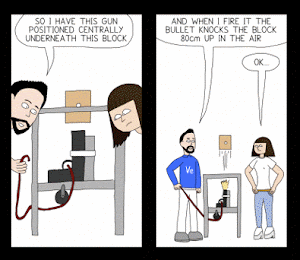A real-life experiment[*] (illustrated in webcomic format at right[**]) shot a bullet upwards that embedded in a wood block, and the block (with the bullet inside) was free to move upwards to a maximum height of 0.80 m.

An independent analysis[***] assuming a bullet mass of 2.3×10–3 kg and a block mass of 0.222 kg estimates that the bullet speed just before entering the block was 384 m/s. Ignore friction, drag, and external forces for this brief collision. Determine whether this claim for the bullet's speed is plausible. Show your work and explain your reasoning using properties of collisions, energy (non-)conservation, and momentum conservation.

[*] Derek Muller (Veritasium), "Bullet Block Explained!" youtu.be/BLYoyLcdGPc.
[**] Ben Dickson (Random Perspective Comic), "Problem Solving," randomperspective.com/comic/80/.
[***] John Rowe, "Bullet in the Block Mystery," compadre.org/IVV/docs/Bullet-Block_Recitation_Tutorial.pdf.

• p:
Correct. Momentum is conserved when bullet embeds in block in a perfectly inelastic collision, then as the bullet-embedded block travels upwards, the translational kinetic energy is converted into gravitational potential energy, and determines that the independent analysis claim of the bullet's initial speed is plausible (to two significant digits) or implausible (due to the very small discrepancy in comparing values) by either:
1. working backwards to determine expected initial speed of bullet, and compares it to the given value of 384 m/s;
2. works forwards to determine expected maximum height of bullet-embedded block, and compares it to the given value of 0.80 m;
3. comparing speed (or translational kinetic energy) of bullet-embedded block just after the perfectly inelastic collision, with the expected speed (or translational kinetic energy) of the bullet-embedded block to travel upwards to a maximum height 0.80 m.
• r:
Nearly correct, but includes minor math errors. May not have squared translational kinetic energy velocity terms, or simple arithmetic errors, but makes a sound argument based on the numerical values resulting from these errors.
• t:
Nearly correct, but approach has conceptual errors, and/or major/compounded math errors. Certain parameters are misplaced or misidentified, but at least successfully applied momentum conservation to the perfectly inelastic collision of bullet and block, but mechanical energy conservation of upwards-moving bullet-embedded block has multiple issues.
• v:
Implementation of right ideas, but in an inconsistent, incomplete, or unorganized manner. At least one conservation law is correct (or nearly correct), other is garbled.
• x:
Implementation of ideas, but credit given for effort rather than merit. No clear attempt at applying conservation laws.
• y:
Irrelevant discussion/effectively blank.
• z:
Blank.
Sections 70854, 70855, 73320
Exam code: midterm02veR1
p: 26 students
r: 5 students
t: 7 students
v: 16 students
x: 10 students
y: 2 students
z: 0 students

A sample "p" response (from student 9178) comparing the calculated initial speed of the bullet (given the maximum height of the bullet-embedded block) with the given value of 384 m/s:A sample "p" response (from student 0000) finding the predicted height that the bullet-embedded block would rise up to, comparing it to the observed 0.80 m height:A sample "p" response (from student 3918) comparing the translational kinetic energy of the bullet-embedded block (just before rising upwards after the collision) with the gravitational potential energy at its highest point:A sample "p" response (from student 7007) comparing the speed of the bullet-embedded block (just before rising upwards after the collision) with the speed required to make it up to a height of 0.80 m:## 20141125

### Physics presentation: internal energy conservationSigh. This equation. The ubiquitous "Q = m·c·∆T" endlessly recited in chemistry, more than likely without much understanding. And by "understanding," I mean, "physics."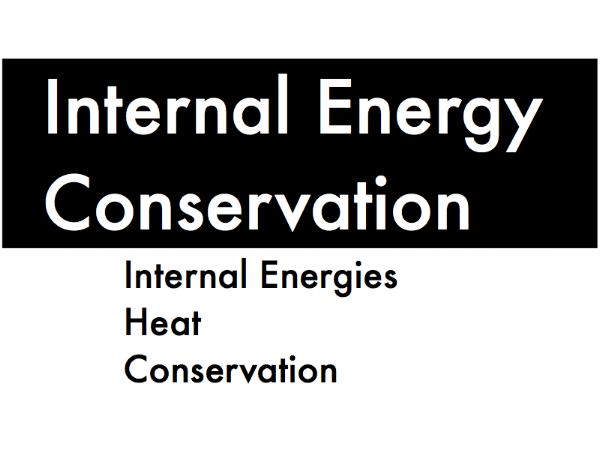Well, now that you've seen that equation, you can't unsee it, so in this presentation we'll instead emphasize the conceptual meaning of heat transfers (the "Q" on the left side of the equality), and of changes in thermal internal energy (the m·c·∆T" on the right side of the equality), and see how this equation works in the context of principles developed earlier in this course for work and mechanical energy conservation.First, introducing internal energy systems, and especially changes in thermal internal energy.Recall that mechanical energy systems such as translational kinetic energy and rotational kinetic energy are concerned with the macroscopic translational motion or rotational motion of an entire object. Thermal internal energy is also concerned with a motion of an object, more specifically the random microscopic motion of the individual atoms and molecules within an object. So for this just-cooked turkey coming out of the oven, it contains a large amount of thermal internal energy due to the very active microscopic motion of its atoms and molecules.The total thermal internal energy of an object's microscopic atomic and molecular motion depends on its mass m and its temperature T (measured in kelvins), and is expressed as joules (chemists use "calories" or "Calories" for energy--be sure to ask them why they keep using those non-Système International units). A low temperature object has very little thermal internal energy, and higher temperature it has, the more thermal internal energy it has. The intensive property of different materials (due to its atomic and molecular make-up) related to its thermal internal energy is the "specific heat capacity," in units of J/(kg·K).

(Note that while your textbook explains in detail what thermal internal energy is, it does not actually define a symbol for thermal internal energy, so for our purposes we'll use "Etherm.")

Instead of finding out how much thermal internal energy Etherm an object has, often we are more concerned with its initial-to-final change ∆Etherm, which is the final amount of thermal internal energy minus the initial amount of thermal internal energy. Notice how the common factors of mass m and specific heat capacity c are pulled out, such that the amount of change in thermal internal energy is determined by the ∆T change in temperature.Recall that mechanical energy systems such as gravitational potential energy and elastic potential energy are concerned with the storage of energy due to height of an object in a gravitational field, or due to the compression or stretching of a spring or an elastic material from its equilibrium state. Bond internal energy is concerned with the storage of energy, but stored in the spring-like bonds between the individual atoms and molecules in solids and liquids.

During vaporization (liquid turning into gas) or melting (solid turning into liquid), these bonds are broken, freeing up individual atoms or molecules. Bond internal energy increases during these processes, much like the elastic potential energy of a spring increasing as it stretches more and more, because the bonds between atoms and molecules must be stretched further apart in order to "disconnect" them from each other.

During condensation (gas turning into liquid) or freezing (liquid turning into solid) bonds begin to form between the individual atoms or molecules. Bond internal energy decreases during these processes, much like the elastic potential energy of a spring decreasing as it stretches less, because the distances between atoms and molecules must brought be closer together in order to "connect" them to each other.

(While there is a "latent heat" equation to calculate the change in the bond internal energy during phase changes (when bonds are broken or made between individual atoms and molecules), we will focus primarily on changes in thermal internal energies, so only consider situations where there are changes in temperature, but no changes in phase.)Next, heat--the "Q" in the "Q = m·c·∆T" equation.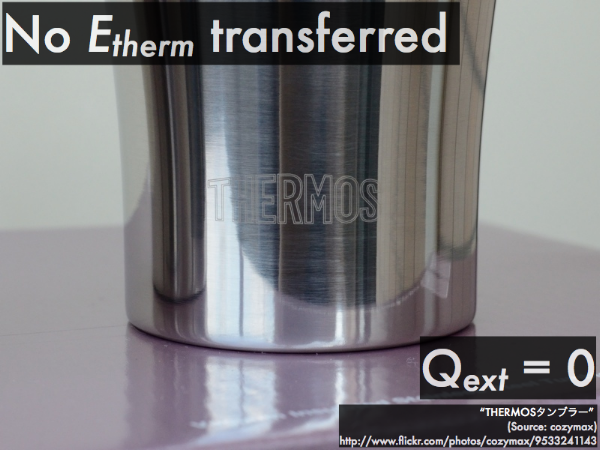Recall that work is the transfer of mechanical energy on the macroscopic level, whether put in by an external agent, or taken away because of friction/drag. Heat, then, is the transfer of internal energy on a microscopic level.

If there is no energy transferred into or out of the thermal internal energy of a system (as with the contents an extremely well-insulated Thermos® bottle), then it is effectively thermally isolated from the environment, and the heat exchanged between the system and the external environment is zero.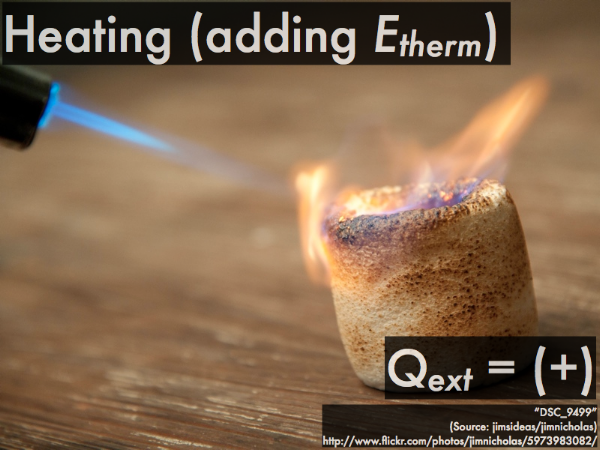If the thermal internal energy of a system increases, its temperature increases, and thus external heat from the environment is positive, being added into the system (as for this blowtorch on a marshmallow).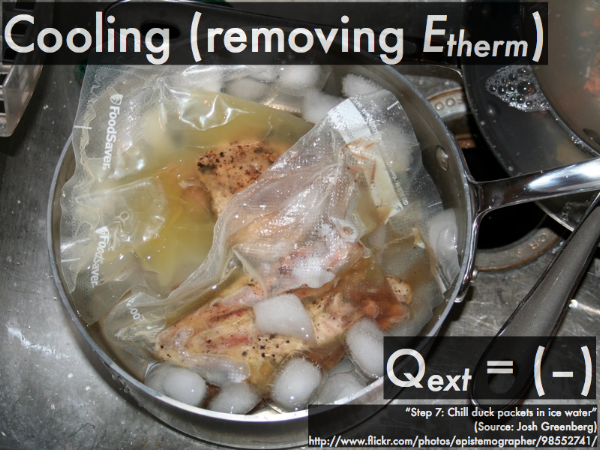If the thermal internal energy of a system decreases, its temperature decreases, and thus external heat from the environment is negative, being removed from the system (as for these sous-vide cooked portions of duck meat being chilled in an ice-water bath).The direction of heat flow is determined by the relative temperatures of two objects interacting with each other--the object at a higher temperature will "heat up" (transfer energy to) an object at a lower temperature. The two objects will stop transferring energy between each other when they attain the same final temperature (such that there is no longer a direction for heat to flow), thus reaching thermal equilibrium.

(Don't ever expect heat to spontaneously flow from a lower temperature object to a higher temperature object--unless you "do work" (expend mechanical energy) to make this happen, which is why it will cost you to run a refrigerator or air conditioner to remove heat from the low temperature contents, and dump it to the warmer environment outside. We'll focus on the "natural" direction of heat flow that occurs between two different temperature objects that thermally interact with each other.)Third, accounting for the transfers between different thermal internal energy systems, and also for the contribution to or taking from these energies by an external source of heat.This is expressed in a format in order to emphasize the parallels with the total mechanical energy conservation equation developed earlier. The transfer/balance equation here shows how the transfer of energy (as heat exchanged by external agents and/or the environment) causes corresponding changes in the thermal internal energies of all objects in our system. Any or all of these thermal energy forms on the right-hand side of the equation can increase or decrease (due to an increase or decrease in temperature), but together all of their changes must add up to the corresponding heat exchange term on the left-hand side of this equation.

When you substitute the individual ∆Etherm terms on the right-hand side of the equation with the equivalent m·c·∆T expressions, then this results in the oh-so-familiar-but-maybe-not-quite-so-meaningless-anymore "Q = m·c·∆T" equation.

Note that in the idealized case that the system is thermally isolated from both external agents and the environment, then the left-hand size of this equation would be zero. Then the individual energy terms on the right-hand side of this equation can then trade and balance amongst themselves, instead of with the outside world.

So now let's see how this transfer/balance equation can be applied to idealized situations where heat gain/loss exchanges with the outside world are negligible compared to the exchanges within a system.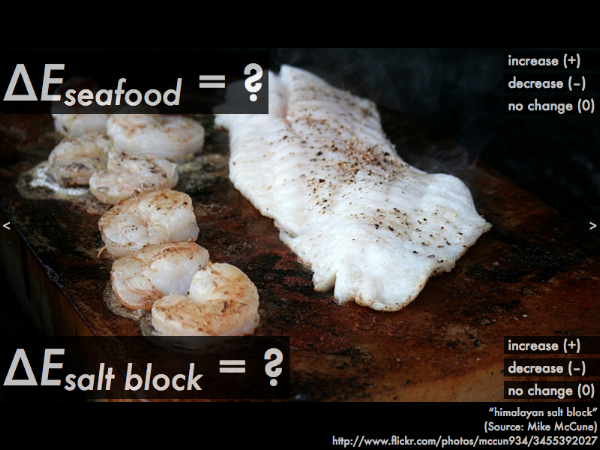Raw seafood is placed on a block of salt that has already been heated up in an oven. The energy contained in the high-temperature block of salt is then transferred to the seafood, cooking it. While it is being cooked, does the internal thermal energy of the seafood increase, decrease, or not change? Does the thermal internal energy of the salt block increase, decrease, or not change?

Assuming that the seafood and salt block system is thermally isolated from the environment (such that Qext = 0), which thermal internal energy experienced a greater amount of change: the seafood, or the block?

As the seafood cooks, its internal thermal energy increases, as the temperature of the food increases. (If you calculated the change in internal energy of the seafood, you would get a positive value, which is consistent with an increase.)

As the salt block cooks the seafood, its internal thermal energy decreases, as the temperature of the salt block decreases. (If you calculated the change in internal thermal energy of the salt block, you would get a negative value, which is consistent with a decrease.)

Since we are assuming idealized situations where heat gain/loss exchanges with the outside world are negligible compared to the exchanges within a system, then there is no heat given off or taken in from the environment, so the left-hand side of the transfer/balance equation is zero:

Qext = ∆Eseafood + ∆Esalt block,

0 = ∆Eseafood + ∆Esalt block,

then we are only left with the changes in the thermal energies of the seafood and the salt block:

0 = ∆Eseafood + ∆Esalt block,

0 = (+) + (–).

Now we can see that amount that the seafood's internal thermal energy increases (where ∆Eseafood is positive) is directly related to the amount that the salt block's internal thermal energy decreases (where ∆Esalt block is negative), in order to equal the zero on the left-hand side of the equation. So the salt block's internal thermal energy "feeds" (or is "transferred to") the seafood's internal energy during this process.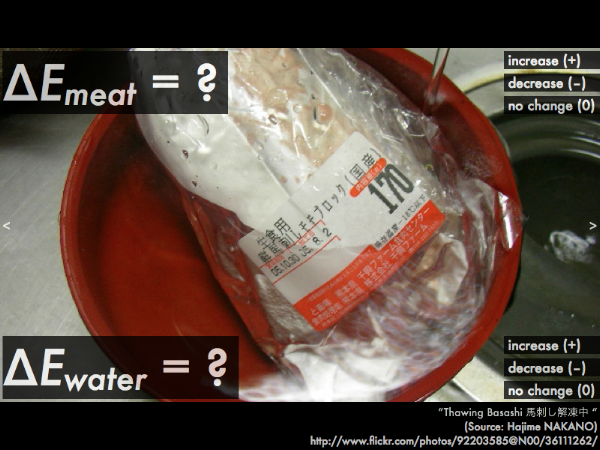Frozen meat is placed in a water bath, in order to defrost it. At the very start of this defrosting process (where the frozen meat just begins to warm up from its below-freezing temperature, and the ice crystals inside have not yet reached the melting point), does the internal thermal energy of the meat increase, decrease, or not change? Does the thermal internal energy of the water increase, decrease, or not change?

Assuming that the meat and water system is thermally isolated from the environment (such that Qext = 0), which thermal internal energy experienced a greater amount of change: the meat, or the seafood?A shot of whiskey is mixed with a pint of beer to make a boilermaker. Assuming that the whiskey and beer have approximately the same temperature before they are mixed together, does the internal thermal energy of the shot of whiskey increase, decrease, or not change? Does the thermal internal energy of the pint of beer increase, decrease, or not change?

Assuming that the whiskey and beer system is thermally isolated from the environment (such that Qext = 0), which thermal internal energy experienced a greater amount of change: the whiskey, or the beer?

(If you haven't noticed the type of vocabulary used in this presentation, we are deliberately avoiding the confusion between "hot" (in terms of high temperature, high thermal internal energy objects) and "heat" (thermal energy transferred between objects).)

## 20141122

### Physics presentation: soundApparently the ultimate test of an impressive car sound system is not how loud it is, but is the subwoofer powerful enough to vibrate the air and shred a phonebook? (Video link: " MD style Paper Shredder !!!!.")In a previous presentation, we introduced wave parameters and standing waves along strings. Here we will extend these ideas to sound waves traveling in air.First, sound wave parameters.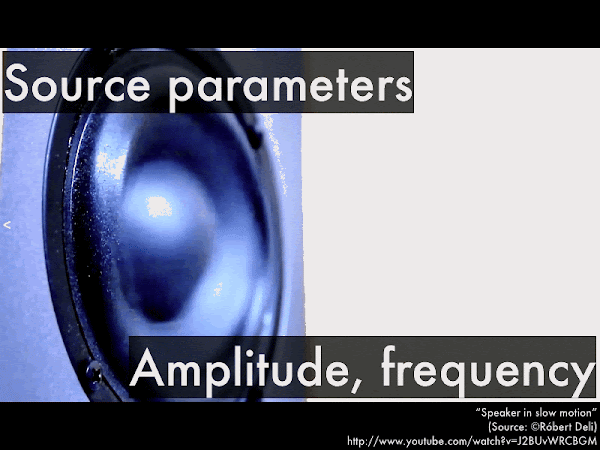The amplitude and the frequency of a sound wave is set by the source (here, a speaker cone) which will vibrate in and out, displacing the air in front of it.The amplitude of the speaker's in-and-out vibrations is related to the "loudness" of a sound--small amplitude vibrations barely displace the air molecules in front of the speaker from their nominal positions, while large amplitude vibrations will greatly displace the air molecules from their nominal positions. (The amount that the air is displaced will decrease as a sound wave spreads outwards from a source--thus making sounds "quieter" as you move further away from a source--but for the purposes of this presentation we'll ignore the spreading out of sounds.)The frequency of the speaker's in-and-out vibrations is related to the "pitch" of a sound--we perceive different frequency sounds in the range from 20 Hz (very low bass notes) up to 20,000 Hz (extremely high treble notes). These are nominal values, and typically the high end is lost due to age or unsafe sound exposure. (There can be sounds with frequencies lower than 20 Hz, if the source vibrates slowly enough--we humans can't hear these infrasound frequencies, but elephants can send out and receive these type of sounds over great distances to communicate with each other. Likewise if a source vibrates quickly enough, it will produce sounds with frequencies higher than 20,000 Hz--we humans can't hear these ultrasound frequencies, but bats can send out and receive these type of sounds, typically for navigating and preying on insects.)The next sound wave parameter is the speed that it propagates through air. Here we can see the spherical wavefront of the gun blast sound emitted from both the muzzle (and earlier wavefronts from the rear of the barrel). Note that the bullet is traveling faster than the speed of sound, resulting in a cone-shaped shock wave. More on that later.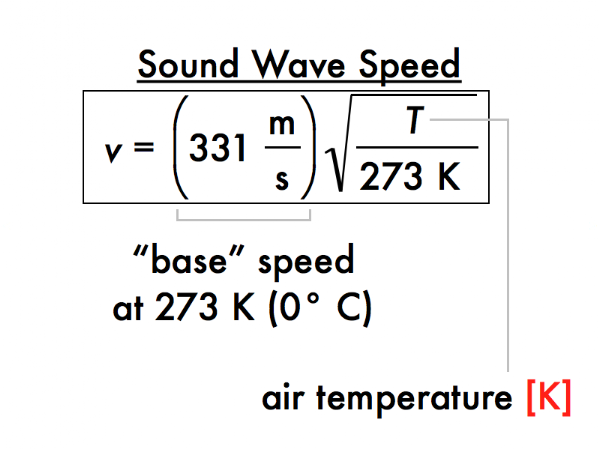The speed of a sound wave in air depends approximately on the square root of the absolute temperature (in kelvin, not in Celsius!), and at 273 K (0° C) would have a speed of 331 m/s, and at "room temperature" (according to some) of 293 K (20° C) sound waves would have a speed of:

v = (331 m/s)·sqrt((293 K)/(273 K)) = 342.91026079 m/s,

or to three significant figures, 343 m/s. (Some may define room temperature as high as 25° C, but apparently physicists like it a little cooler than some other people.) Essentially sound travels faster through warmer air, and slower through cooler air (neglecting changes in pressure). These sound waves are fast, but bullets and jets can certainly move faster than these speeds.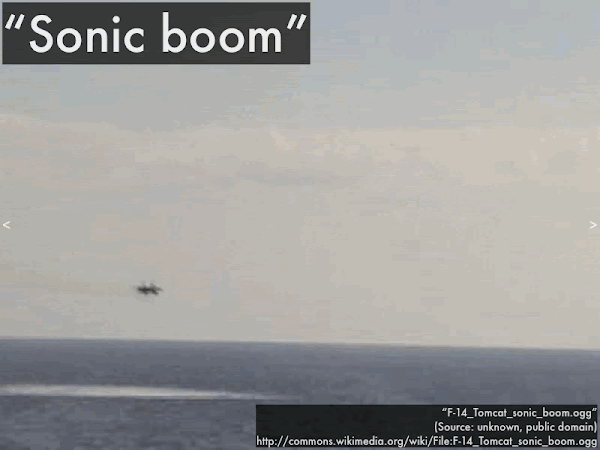Here, an F-14 Tomcat moving faster than the speed of sound creates that characteristic cone-shaped shock wave, which to an observer sounds as a sonic boom. (Video link: "F-14_Tomcat_sonic_boom.ogg.")The speed of a sound wave is set by the temperature of the medium (air), but the frequency of the wave is set by the source. The resulting spatial repeat interval is the wavelength, which is the distance between the "crowding" wavefronts. Note that while a single air molecule (highlighted in yellow) just moves back-and-forth (at the average random speed of an ideal gas at that temperature), the wavefronts of a wave travel from left-to-right at the speed of sound.(This is a review of our previous discussion of one-dimensional rope and string waves.) Note the hierarchy of these wave parameters. Since the wave speed is determined by properties of the material it travels through (independent of the source), and the frequency is determined by the source (independent of the medium), these are said to be independent wave parameters. In contrast, the wavelength of the wave is dependent on both the independent speed and frequency parameters. Algebraically there is nothing wrong with expressing this relation as v = λf and f = v/λ, as long as you recognize that the dependency of λ doesn't change.The speaker on the left oscillates back-and-forth as the source of a sound wave that travels left-to-right along this section of air. The top case is where the speaker oscillates back-and-forth with a certain frequency and a small amplitude, while the bottom case is where the speaker oscillates back-and-forth with the same frequency and larger amplitude. Which wave travels with the faster speed? Which wave has the longer wavelength?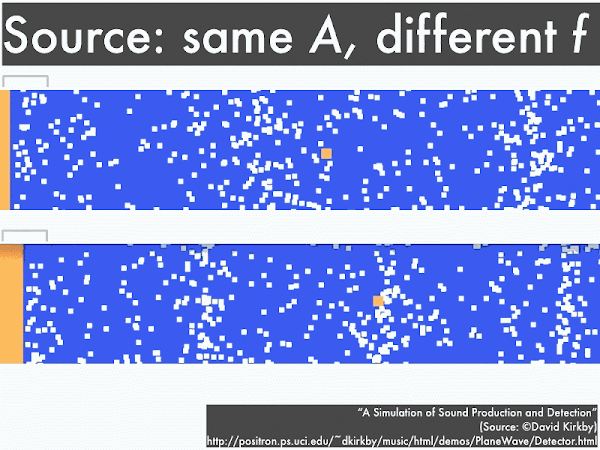Here both speakers oscillate back-and-forth with the same amplitude, but the top case has a lower frequency and the bottom case has a higher frequency. Which wave travels with the faster speed? Which wave has the longer wavelength?In this setup, the a speaker oscillates back-and-forth with a given frequency and amplitude, creating the waves in the first section at left. As the wave travels from left-to-right, it is then transferred to the second section that allows the wave to travel at a much slower speed (perhaps due to a cooler air temperature, or a different gas density). Along which section does the wave have a higher frequency? Along which section does the wave have a longer wavelength?Now we'll briefly extend our previous discussion on standing waves on strings to sound standing waves in pipes.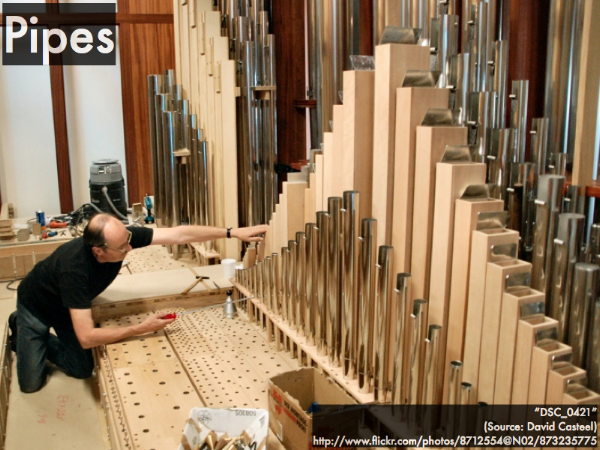Sound waves can be "trapped" inside pipes of various lengths, with either open and/or closed ends, and if a sound wave matches the resonant frequency of the pipe, then the air inside will move back-and-forth in a coordinated manner--a "standing wave."We can regard a pipe with both ends closed, or a pipe with both ends open as a "symmetric" system. If they have the same length, then the air molecules will resonate with the same fundamental frequency (in this animation, approximately 1 Hz). Note that while the standing waves have the same frequency for both pipes, the closed-closed pipe has an antinode in the middle, where air molecules slosh back-and-forth, while there are nodes at either end, where air molecules are "trapped" because they cannot move into (against) the closed ends.

In contrast, the open-open pipe has antinodes at either end, as air molecules are free to slosh back-and-forth, being exposed to the atmosphere, while there is a node in the middle, where air molecules are "trapped" because of the motion at either end.

Because the distance between consecutive nodes and antinodes in these pipes is proportional to the wavelength of the standing wave, the fundamental frequency would be the same for either closed-closed or open-open pipes.For a closed-closed or open-open symmetric pipe, the frequency at which a source of sound waves would resonate within this pipe are multiples of the fundamental frequency f1, which depends on the wave speed v of sound (343 m/s for "room temperature"), and the length of the pipe.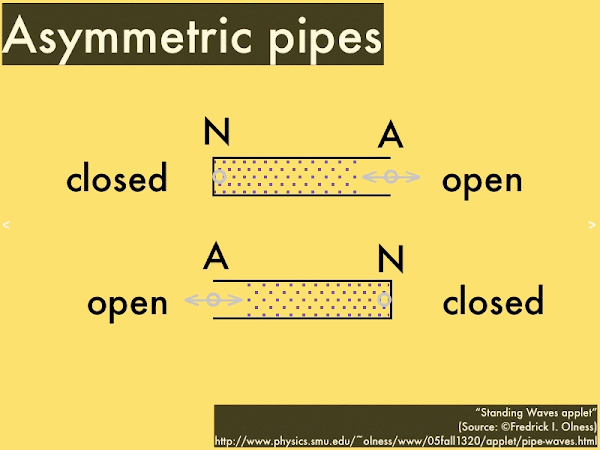We can regard a pipe with one end open, while the other end is closed (or vice versa) as a "symmetric" system. If they have the same length, then the air molecules will resonate with the same fundamental frequency (in this animation, approximately 2 Hz). Note that while the standing waves have the same frequency for both pipes, the open end has an antinode, where air molecules exposed to the atmosphere slosh back-and-forth, while the closed end has a node, where air molecules are "trapped" because they cannot move into (against) the closed ends.

(Because the distance between consecutive nodes and antinodes in these pipes is proportional to the wavelength of the standing wave, the fundamental frequency would be the same for either closed-open or open-closed pipes. Note for a given length of pipe, the asymmetric systems have a longer (bigger value) node-antinode spacing than the symmetric systems, and thus an asymmetric system would have a lower (smaller value) fundamental frequency (in this animation, approximately 2 Hz) than the symmetric system.)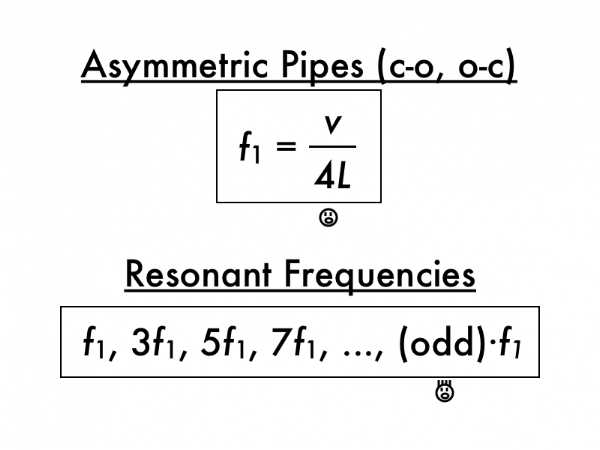For a closed-open or open-closed asymmetric pipes, the fundamental frequency is lower than a comparable symmetric pipe (note the factor of 4 instead of 2 in the denominator), and resonant frequencies are all odd multiples of the fundamental frequency (rather than integer multiples). How very, very...odd. But this follows from the asymmetry of closed-open and open-closed pipes, such that even standing wave frequency multiples are not allowed.Let's close out with some trombone playing, which can be approximated as a symmetric pipe (the bell end is open to the air, while the mouthpiece end is open to your lungs). In order to play different notes on a trombone is to buzzing your lips, not just at any arbitrary frequency, but only at multiples of the fundamental frequency in order to resonate the air along the pipe. Higher resonant frequency notes can be accomplished either by changing the length L of the trombone by shortening the slide... (Video link: "How to play the Trombone B Flat Major Scale.")...or by keeping the position of the slide fixed (such that L is constant), and buzzing your lips to match higher resonant frequency notes. (Video link: "Trombone B Flat 7 Octaves Attempt.") This takes a fair amount of manual and lip coordination, so keep that in mind the next time you are subjected to a novice trombone player practicing the scales.